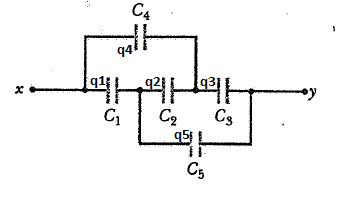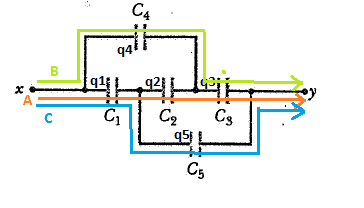# Equivalent capacitance of a system from potential difference

0 votes
240 views

Calculate the equivalent capacity of the capacitor system between points x and yI'm asking about this problem because I know how to calculate $V_x-V_y$, but I don't know how can I extract the equivalent capacity from there.
Using Kirchoff Laws I get the following equations

1. $$V_x-V_y=q_1/C_1+q_2/C_2+q_3/C_3$$
2. $$V_x-V_y=q_4/C_4+q_3/C_3$$
3. $$V_x-V_y=q_1/C_1+q_5/C_5$$
4. $$0=-q_1/C_1-q_2/C_2+q_4/C_4$$
5. $$0=q_2/C_2+q_3/C_3-q_5/C_5$$

($1,2,3$ express $V_x-V_y$ through all the possible paths from $x$ to $y$ I can take, while $4,5$ are loop laws for the two loops).

I can solve for $V_x-V_y$, but then here it comes the doubt.

As said I have three different ways to go from $x$ to $y$ (the first three equations are there because of that), I highlighted them in the picture (path $A$ is equation $1$, path $B$ is equation $2$, path $C$ is equation $3$)So can I express the equilvalent capacitance of the system as the ratio of the charge met following the particular path and $V_x-V_y$? That would be

$$C_{equivalent}=(q_1+q_2+q_3)/V_x-V_y=(q_4+q_3)/V_x-V_y=(q_1+q_5)/V_x-V_y$$

This does not look correct at all, but it is the only reasonable way to use the potential difference to calculate capacity.

So I ask: what is the right way to link $V_x-V_y$ to $C_{equivalent}$? That is : how to calculate $C_{equivalent}$ knowing $V_x-V_y$?

I would like to use this method (using $V_x-V_y$) and not other ones (if there are any) because I would like to understand how it should work, so please help me figure out the link between $V_x-V_y$ and $C_{equivalent}$ in cases like this one.

asked Nov 2, 2016
edited Nov 9, 2016
P.S: Latex not rendering.
Latex has now been configured :)

## 1 Answer

2 votes

Best answer

One of the problems is that for a capacitor like $C_2$ you can't be sure which way it's polarity is going to end up. It will depend upon the values of the other capacitors around it, which create voltage dividers. What you can do is write mesh equations for the charges (as opposed to currents). When some hypothetical voltage is placed across xy then there will be a brief transient time during which currents flow to move the charges to their steady state locations. Assign "mesh charges" to each loop and write KVL. Solve for the mesh charge associated with the voltage supply loop.KVL will sort out all polarity issues for you, all you need to do is respect the mesh charge directions while writing the equations.

answered Nov 2, 2016 by (160 points)
edited Nov 9, 2016 by Einstein